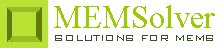Diffusion of molecules in a resting fluid

dcoef      diffusion coefficient of solute [m2/s]
time        time passed after the start of diffusion [ms]
sel          number denoting the selected result.
Use 2 for diffusion length

Notes

An important problem in microfluidic diffusion is the spreading of solute molecules in a solvent that is at rest. It is interesting to know how far and how much the molecules have spread after any given time.

The two problems under consideration are limited point source diffusion and constant planar source diffusion. In limited point source diffusion, a fixed number of solute molecules (say a drop of ink) is injected as a spot into a resting solvent. An instant after that, the spot starts to spread and the solute  concentration at the insertion point reduces. The calculated diffusion length is a statistical approximation of how far the molecules have spread after any given time in one dimension. It is also the "width" of the distribution. In constant planar source diffusion, the solute molecules are not limited in number and a constant influx of solute molecules is maintained at one of the boundary surfaces.

The plot shows the distribution of the solute concentration after the given amount of time. It shows the normalized concentration of solute molecules along an unidirectional axis pointed away from the point of insertion for limited source and constant source diffusion. The constant source diffusion will have constant concentration at the boundary surface (normalized conc. = 1 at x = 0 , t > 0) while it drops off for limited source diffusion immediately after the start of diffusion. A linear or logarithmic plot can be selected by right clicking the plot graph button.

Assumptions

-The velocity field of the solvent is zero.
-The fluids has constant viscosity.